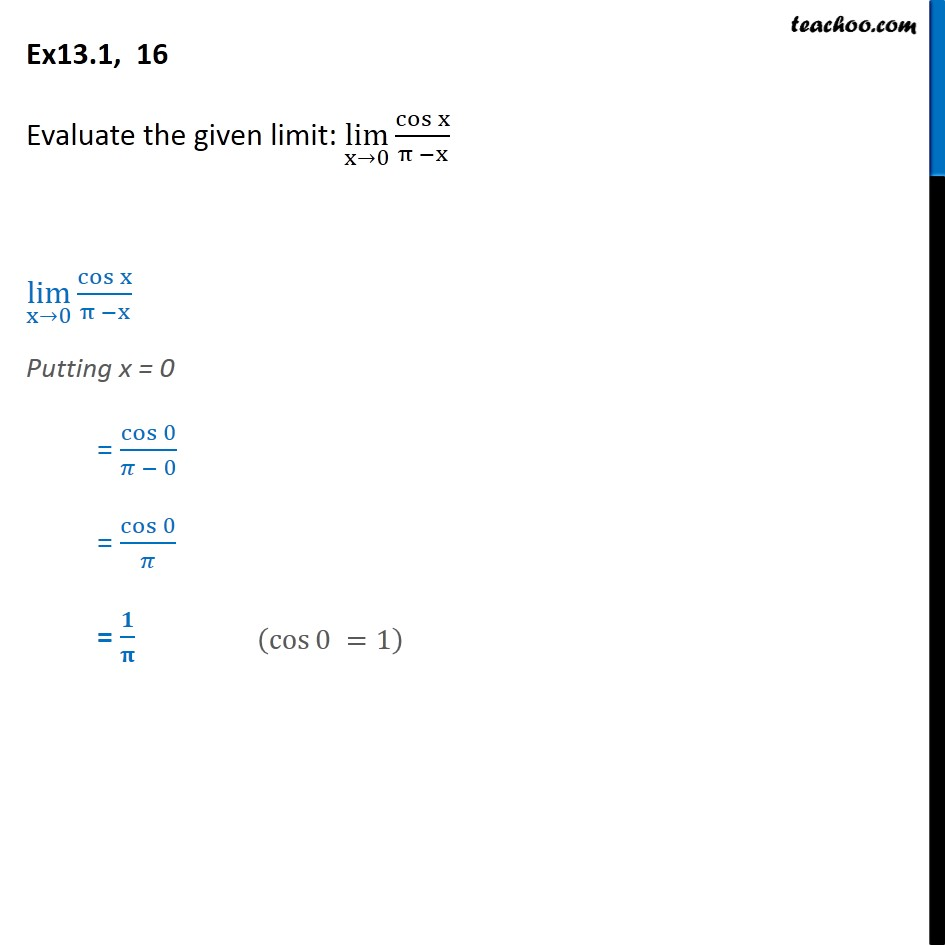1. Chapter 13 Class 11 Limits and Derivatives
2. Serial order wise
3. Ex 13.1

Transcript

Ex 13.1, 16 - Chapter 13 Class 11 Limits and Derivatives - NCERT Evaluate the given limit: lim (x→0) cos⁡ x / (π − x) lim (x→0) cos ⁡x / (π −x) Putting x = 0 = cos⁡0/(𝜋 − 0) = cos⁡0/𝜋 = 𝟏/𝛑 (cos 0 = 1)

Ex 13.1